Courses

# Starting And Braking Of DC Series Motors Electrical Engineering (EE) Notes | EduRev

## Electrical Engineering (EE) : Starting And Braking Of DC Series Motors Electrical Engineering (EE) Notes | EduRev

The document Starting And Braking Of DC Series Motors Electrical Engineering (EE) Notes | EduRev is a part of the Electrical Engineering (EE) Course Electrical Machines.
All you need of Electrical Engineering (EE) at this link: Electrical Engineering (EE)

Starting and Braking of DC Series Motors
(Refer Slide Time: 00:24)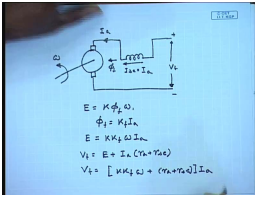In the last class, we have derived the torque speed characteristics of a DC series motor. As in a DC series motor the field winding is connected in series with the armature, if we neglect armature reaction and saturation then we can derive a analytical expression for the torque speed characteristics as follows. The generated voltage E equal to K phi f this is phi f, omega, where phi f equal to from K f I a, and therefore E equal to K K f omega I a also V t equal to E plus I a into r a plus r s e or V t equal to K K f omega plus r a plus r s e into I a.

(Refer Slide Time: 02:23)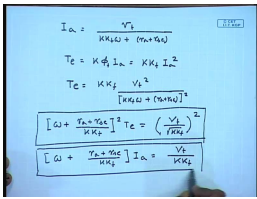You can write I a as V t divided by, torque is given by K phi f I a equal to K K f I a square therefore, torque equal to K K f into V t square divided by K K f omega plus r a plus r s e whole square. Now, this can be written as omega plus r a plus r s e divided by K K f whole square into T e equal to V t divide by square root K K f whole square this is the analytical expression of the torque speed characteristics of a DC series motor when saturation is neglected. Now if we plot this, the current speed expression can also be determined in a similar manner. This is the current speed characteristics, now if you plot these, both of them.

(Refer Slide Time: 04:58)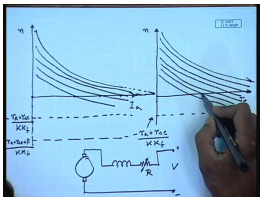A current speed is a rectangular hyperbola with the y axis and the axis at n equal to minus r a plus r s e by K K f as asymptotes the same thing for the torque speed characteristics. Obviously at start that is at n equal to 0, the current and the torque will be determined by the intersection of this curve with the x axis, in normal situation this current will be almost an order of magnitude larger than the rated current of the machine, so will be the torque. So, we have seen for DC shunt machine also similar situation arises, therefore it is necessary to limit the starting current even in case of a DC series motor and that can be done by putting additional resistance in series with the armature.

Now, if we put additional series resistance then this asymptote line goes down this is because of minus r a plus r s e plus R divided by K K f and this characteristics also lowers for different values of R you can get different characteristics like this. So, it is possible to restrict the starting current at a desirable value by choosing this resistance external resistance judiciously, same thing will happen to torque speed characteristics; however, we see that as the resistance is increased the current through the machine reduces and so does the torque therefore, the machine takes longer time to start. In order to maintain a almost constant starting torque in that case this resistance should be continuously variable which is difficult to achieve.

(Refer Slide Time: 09:24)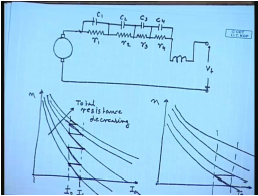So, we use sectionalized resistance as in the case of a DC shunt motor for starting a DC series motor also, like this. These are contactors, which can short this sectionalized resistance. So, if we plot the current speed characteristics and the torque speed characteristics with different resistances in series. For different resistances these are with resistance decreasing, total resistance. So, suppose we start with the maximum resistance and this will be the starting current and as the machine speed up this is the maximum allowable current let us say I 1. As the machine speed up the current reduces so does the torque and at this value let us say we short the first resistance.

Since the speed cannot change immediately, it shifts to the next characteristics on a horizontal line and the speed increases further then at some point we short the second resistance. The current again jumps and we keep on doing this till we arrive at the natural characteristics of the machine. So, here also we see that we can arrange sectionalized resistance and gradually reduce them in order to get a almost constant starting current and a starting torque.

(Refer Slide Time: 13:27)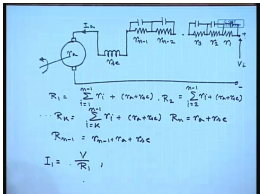How do we calculate these resistances then in case of a DC series motor, for that let us define certain values, this is the field winding these are several resistances. So, this resistance is r s e armature resistance is r a. Let us say this is r n minus 1, r n minus 2, r 3, r 2, r 1 and these are the shorting contactors let us say we want to restrict the current between the limits I 1 and I 2. Let us also define R 1 to be r i plus r a plus r s e, R 2 to be in this manner, R k to be and R n will be nothing but r a plus r s e and R n minus 1 will be equal to r n minus 1 plus r a plus r s e. From the diagram, we have drawn earlier we can write I 1 equal to at start all the resistances are in circuit. So, just when the voltage is applied the current should be r one and the speed at that time is zero. So, I 1 equal to V divided by R 1.

(Refer Slide Time: 17:07)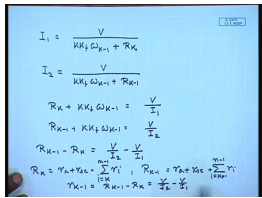In fact, for any resistance section k, we can write I 1 equal to V divided by K K f omega K minus 1 plus R K, where R K is given by this expression, that is just when the R K minus 1. Resistance has been shorted the speed was at omega K minus 1 just when one resistance has been shorted and the new resistance has become R K, the current is I 1. In which case, we can also write I 2 which was the current just before the resistance was shorted is given by V divided by K K f, it has to be same because omega cannot change instantaneously.

R K minus 1 therefore, R K plus K K f omega K minus 1 equal to V divided by I 1 and R K minus 1 plus K K f omega K minus 1 equal to V divided by I 2. So, R K minus 1 minus R K equal to V divided by I 2 minus V divided by I 1, but R K equal to r a plus r s e plus sigma i equal to k to n minus 1 r i, whereas, R K minus 1 equal to r a plus r s e plus sigma i equal to k minus 1 to n minus 1 r i. So, small r of k minus 1 equal to R of K minus 1 minus R of K equal to V divided by I 2 minus V divided by I 1. So, each of these resistances are equal and of the magnitude V divided by I 2 minus V divided by I 1.

So, this is how we can find out the resistances, sectional resistances for starting the DC series motor of course, we have to bear in mind that, this we have done assuming that the saturation is negligible. If we consider saturation then there will be some variation in this resistance value. Just as it is important to restrict the current in a DC series motor during starting the same thing applies for breaking also. Now, breaking is slightly more involved in case of DC series motor as we will see.

(Refer Slide Time: 21:01)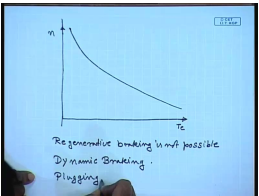The torque speed characteristics of a DC series motor looks somewhat like this. It does not have a natural no load speed because at no load the speed tends to become dangerously high. So, as such regenerative breaking is not possible. However we will see how we can achieve regenerative breaking by using a chopper later on; however, other two method of breaking that is dynamic breaking and plugging is possible.

(Refer Slide Time: 22:40)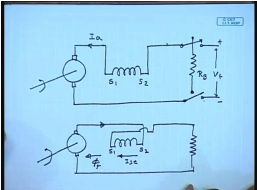Let us see, if how we can do dynamic braking of a DC series motor. What happens during motoring mode the machine is connected to the supply and the armature current flows into the motor. If the machine has to operate in the braking region must operate as a generator and current must be fed to the breaking resistor; that means, the direction of current must reverse, but in a series motor the problem is if the armature current reverses then the field flux also reverses. So, the breaking will not takes place, if we simply change over.

It is also necessary therefore, while braking to also reverse the field winding that is during breaking the connection has to change to the following. The direction of rotation remains same and with a reverse direction of current, now the current will still flow from S 2 to S 1 and the flux will still be in the same direction.

(Refer Slide Time: 25:29)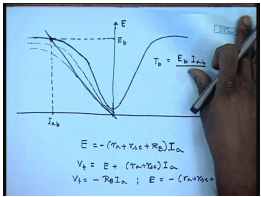So, the polarity of the induced e m f will not change. Graphically we can show it like this, if this is the magnetization characteristics of the DC series motor then when we want to do regenerative breaking and reverse the field winding then the same magnetization characteristics will be obtained from negative current now.

And since the direction of current will change now, the load line equation will be E equal to r a plus r s e, minus, or in other words if we keep the armature current direction same then V t will still be equal to E plus r a plus r s e into I a, but V t equal to minus R B I a, hence E equal to minus of r a plus r s e plus R B into I a, which is again a straight line which intersects the breaking characteristics the O C C during breaking at this point. This will be the value of I a, which shows that I a is negative; that means, the current reverses and this will be the induced voltage. The breaking torque T b will be E b into I a b by omega.

If should be noted that during breaking the series motor operates as a self excited series generator, hence it will have it is self excitation critical resistance and critical speed. So, as the machine speed goes down the O C C will also decrease and at some time it will become tangent to the load line, if the breaking resistance remains same then after some speed the self excited series generator will fail to excite and hence the breaking torque will also reduce drastically. This can be so there should be a method for varying the breaking resistance as the machine slows down.

(Refer Slide Time: 30:20)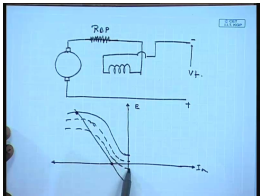This can be done with the help of a chopper as we will see, but before that, let us see how we can do plugging, plugging can be done is a similar manner. Here also we need to reverse the connection of the field winding, but instead of connecting a braking resistance now, this is connected to a voltage of opposite polarity. So now, this will be the magnetization characteristics and the load line which now the applied voltage is not zero, but a negative value.

So, this will be the load line hence, this will be the operating point. We see that for plugging the value of the breaking torque at zero speed is not zero. So, there is a possibility that the machine will start rotating in the opposite direction of course, the current can be very large hence it will be usually necessary to put additional resistance in series with the armature while resulting to plugging. Even here as the machine speed decreases the plugging torque will decrease and it will be desirable to be able to vary the series resistance.

(Refer Slide Time: 32:49)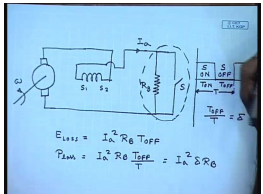This can be done with the help of a chopper. Let us see, we know that a chopper consists of a two features. So, let us first consider dynamic braking, here also it will be necessary to, let us, this is the normal dynamic braking resistor and in order to vary it connect switch across it. A controllable switch S, where the S is turned on and turned off at a constant duty cycle or variable duty cycle at a constant frequency, this is the S on, S off.

Now, there will be power loss, this is the armature current I a there will power loss in R B only when the switch S is off. So, what is the total energy loss? So, this is the time period T, this is the period of T on and T off. So, the energy loss over one cycle in R B equal to I a square R B into T off. So, average power loss equal to I a square R B into T off by T, if I call this to be delta the period over which power loss takes place then this is equal to I a square delta R B.

(Refer Slide Time: 36:36)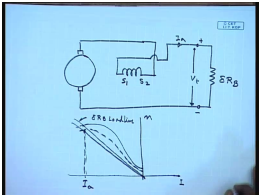So, this entire arrangement of a fixed resistance and a switch now appears as if we have connected a variable resistance R B, delta R B. Here we have connected a variable resistance delta R B, since as the machine slows down the O C C drops, but at the same time the delta can be varied also. So, that the breaking current and hence the torque remains almost constant. So, with a chopper it is possible to maintain a constant breaking current during dynamic breaking. More importantly it is possible to achieve regenerative breaking using choppers.

(Refer Slide Time: 39:05)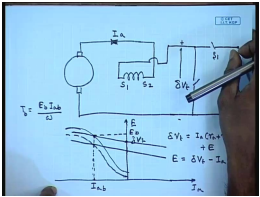So, for that again we will connect the series machine to a chopper with the field reversed, if this switch is turned on at a duty ratio of delta we know that the voltage here will be delta V t. Now, since we have reversed the field winding. Now, for negative current the generated E will be positive with the chopper it was assumed positive current direction to be this, we can write delta V t equal to I a into r a plus r s e plus E. Or E equal to delta V t minus I a, r a plus r s e. So, this is the position delta V t, we can always get a straight line like this. More importantly as them so it is possible to choose a value of delta, that there is a intersection of this inverse O C C, that is O C C for negative current and the load line. So, you get a operating point it is at which the series motor will operate and the braking torque will be E b I a b by omega.

As speed goes down the O C C will decrease the delta can be adjusted. So, that the breaking current and hence the braking torque remains more or less constant. This way we can use a chopper to obtain constant torque during either dynamic breaking or regenerative breaking. Now, let us try to solve a few problems relating to DC series motor to explain to as examples of the concept that we have discussed so far.

(Refer Slide Time: 43:05)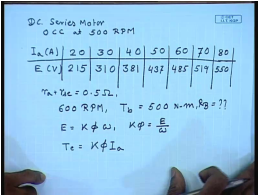Let us take a DC series machine, whose O C C is given by the following data. I a equal to 20 ampere, E is 215 volts, 30 ampere, 310 volts, 40 ampere, 80 ampere, 550 volts. Now, r a plus r s c for this machine equal to 0.5 ohm. This machine is break by dynamic breaking. At 600 R P M we want that at 600 R P M the breaking torque should be equal to 500 Newton meter, what should be the breaking resistance? For this let us first convert the given O C C data in a slightly different format, we know that E equal to K phi omega or K phi equal to E by omega also T e equal to K phi I a even when we consider saturation. So, from the given O C C data we can find out K phi and torque for different currents.

(Refer Slide Time: 46:14)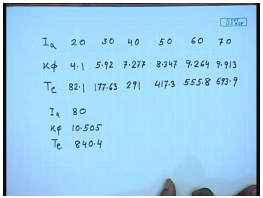So, this table can be converted to the following table, that is I a K phi and T e. So, if you do that and for 20 amperes of I a, K phi becomes 4.1 and T e becomes 82.1. For 30, it is 5.92, 177.63, for 40, 50 and for 80, now if I plot this versus I a, this is K phi and this is T e.

(Refer Slide Time: 48:20)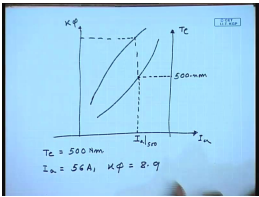This is I a, if I plot will look somewhat like this and T e plot to some extent like this. So, at 600 so if I want torque of 500 Newton meter, we can read out 500 Newton meter from here and the corresponding current I a. So, I a at 500 Newton meter comes out from this diagram. As well as the K phi from here 56 amperes and K phi comes to 8.9.

(Refer Slide Time: 50:29)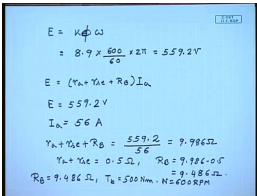Therefore for 600 R P M, E equal to K phi omega is equal to 8.9 into 600 by 60 into 2 pi this comes to 559.2 volts, but in a regenerative braking E equal to r a plus r s e plus and dynamic breaking I a, E equal to this. Therefore, r a plus r s e plus R B comes to equal to 9.986 ohms of which r a plus r s e equal to 0.5 ohm. Hence R B equal to 9.986 minus 0.5 equal to 9.486 ohm.

However it should be understood that, this torque, this resistance R B equal to 9.486 ohm will give a breaking torque T b equal to 500 Newton meter, at N equal to 600 R P M only. If the, as the speed goes down the torque will also go down, hence in order to maintain a fixed breaking torque you will need to vary R B which we have seen can be done using a chopper.

Thank you.

Offer running on EduRev: Apply code STAYHOME200 to get INR 200 off on our premium plan EduRev Infinity!

61 docs|20 tests

,

,

,

,

,

,

,

,

,

,

,

,

,

,

,

,

,

,

,

,

,

;